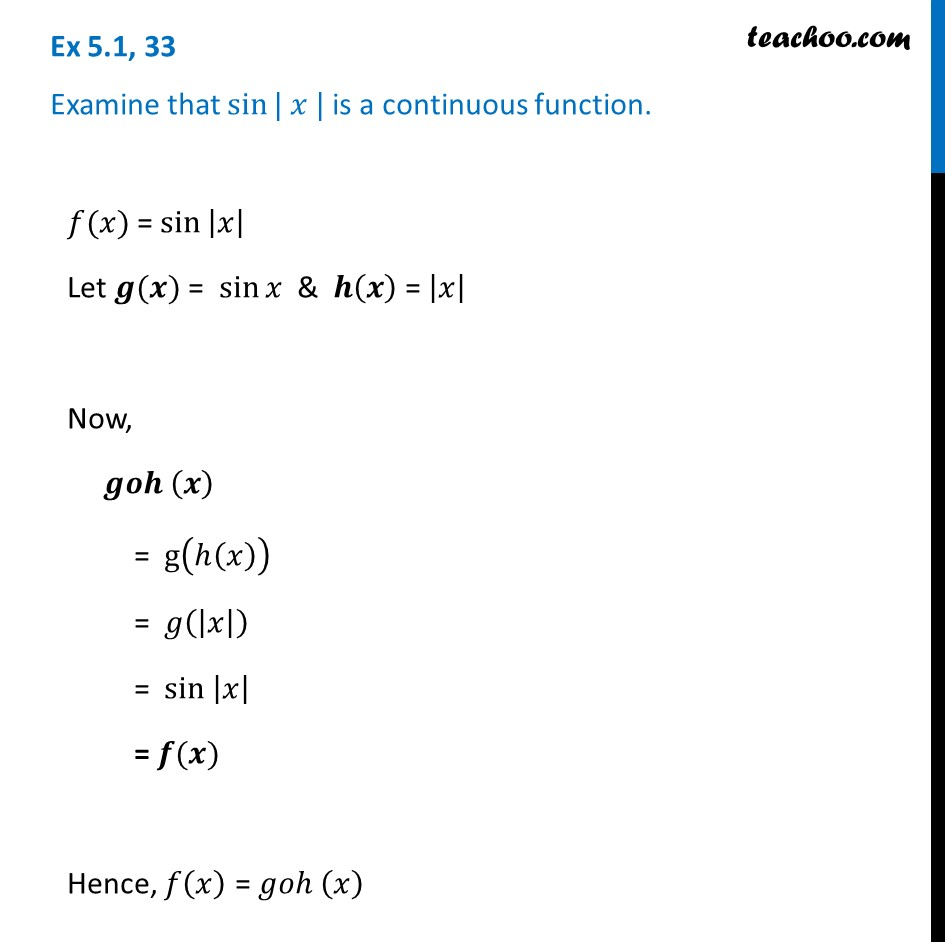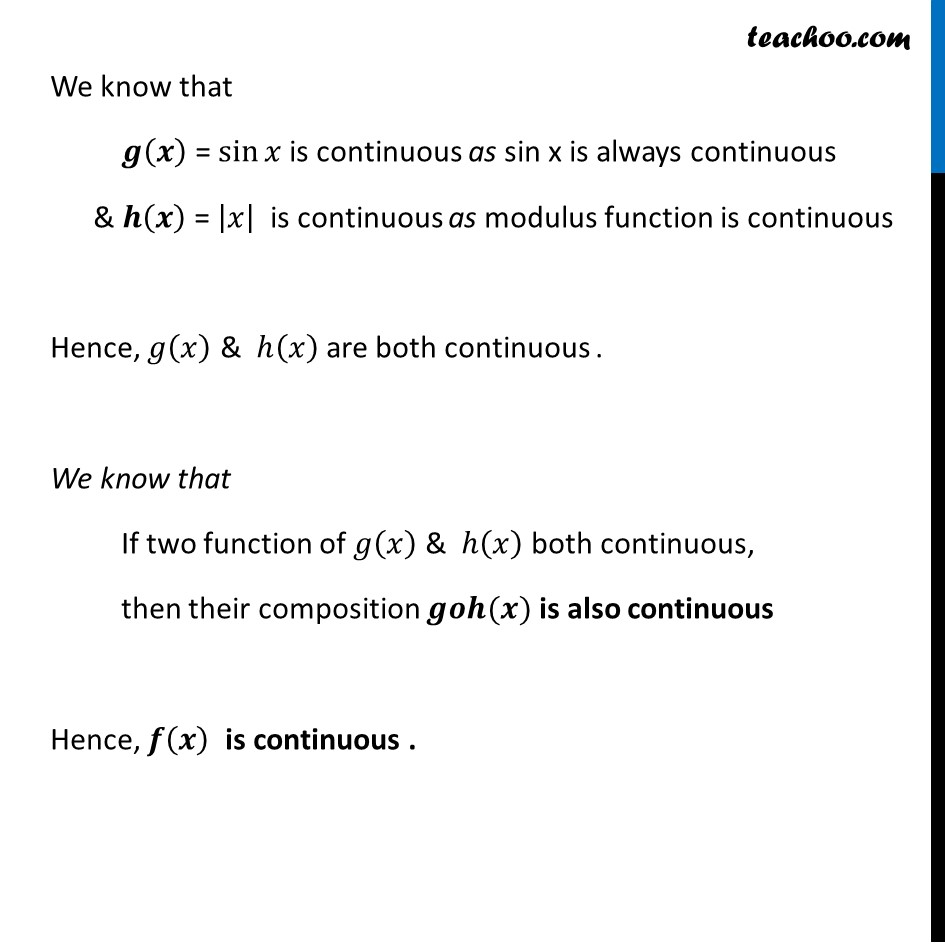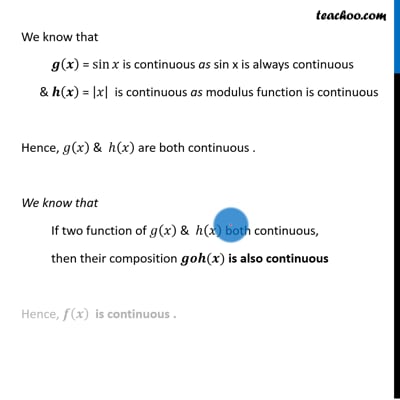Continuity of composite functions

Chapter 5 Class 12 Continuity and Differentiability
Concept wiseThis video is only available for Teachoo black users

Introducing your new favourite teacher - Teachoo Black, at only ₹83 per month

### Transcript

Ex 5.1, 33 Examine that sin⁡| 𝑥 | is a continuous function.𝑓(𝑥) = 〖sin 〗⁡|𝑥| Let 𝒈(𝒙) = sin⁡𝑥 & 𝒉(𝒙) = |𝑥| Now, 𝒈𝒐𝒉 (𝒙) = g(ℎ(𝑥)) = 𝑔(|𝑥|) = 〖sin 〗⁡|𝑥| = 𝒇(𝒙) Hence, 𝑓(𝑥) = 𝑔𝑜ℎ (𝑥) We know that 𝒈(𝒙) = sin⁡𝑥 is continuous as sin x is always continuous & 𝒉(𝒙) = |𝑥| is continuous as modulus function is continuous Hence, 𝑔(𝑥) & ℎ(𝑥) are both continuous . We know that If two function of 𝑔(𝑥) & ℎ(𝑥) both continuous, then their composition 𝒈𝒐𝒉(𝒙) is also continuous Hence, 𝒇(𝒙) is continuous . Given 𝑓(𝑥)= |𝑥| – |𝑥+1|. Here, we have 2 critical points x = 0 and x + 1 = 0 i.e. x = 0, and x = −1 So, our intervals will be When 𝒙≤−𝟏 When −𝟏<𝒙<𝟎 When 𝒙≥𝟎 When 𝒙≤−𝟏 𝑓(𝑥)= |𝑥| – |𝑥+1|. Here, both will be negative 𝑓(𝑥)=(−𝑥) –(−(𝑥+1)) 𝑓(𝑥)=−𝑥+(𝑥+1) " " 𝒇(𝒙)=𝟏 When −𝟏<𝒙≤𝟎 𝑓(𝑥)= |𝑥| – |𝑥+1|. Here, x will be negative, but (x + 1) will be positive 𝑓(𝑥)=(−𝑥) –(𝑥+1) 𝑓(𝑥)=−𝑥−𝑥−1 " " 𝒇(𝒙)=−𝟐𝒙−𝟏 |𝑥| = {█(𝑥, 𝑥 ≥0@−𝑥, 𝑥<0)┤ |𝑥+1| = {█((𝑥+1) , 𝑥+1≥0@−(𝑥+1) 𝑥+1<0)┤ = {█((𝑥+1) , 𝑥≥−1@−(𝑥+1) 𝑥<1)┤ When 𝒙≥𝟎 𝑓(𝑥)= |𝑥| – |𝑥+1|. Here, both will be positive 𝑓(𝑥)=𝑥 –(𝑥+1) 𝑓(𝑥)=𝑥−𝑥−1 " " 𝒇(𝒙)=−𝟏 Thus, our function becomes 𝒇(𝒙)={█(𝟏 𝒊𝒇 𝒙≤−𝟏@−𝟐𝒙−𝟏 𝒊𝒇 −𝟏<𝒙<𝟎@−𝟏 𝒊𝒇 𝒙≥𝟎)┤ Since we need to find continuity at of the function We check continuity for different values of x When x < −1 When x = −1 When −1 < x < 0 When x = 0 When x > 0 Checking continuity Case 1 : When x < −1 For x < −1, f(x) = 1 Since this constant It is continuous ∴ f(x) is continuous for x < −1 Case 2 : When x = −1 f(x) is continuous at 𝑥 =−1 if L.H.L = R.H.L = 𝑓(−1) if lim┬(x→〖−1〗^− ) 𝑓(𝑥)=lim┬(x→〖−1〗^+ ) " " 𝑓(𝑥)= 𝑓(−1) Since there are two different functions on the left & right of −1, we take LHL & RHL . LHL at x → −1 lim┬(x→〖−1〗^− ) f(x) = lim┬(h→0) f(−1 − h) = lim┬(h→0) 1 = 1 RHL at x → 0 lim┬(x→〖−1〗^+ ) f(x) = lim┬(h→0) f(−1 + h) = lim┬(h→0) (−2(−1+ℎ))−1 = lim┬(h→0) (2−2ℎ)−1 = (2 − 2(0)) − 1 = 2 − 0 − 1 = 1 & 𝑓(0) = −1 Hence, L.H.L = R.H.L = 𝑓(0) ∴ f is continuous at x = 0 Case 5: When x > 0 For x > 0, f(x) = −1 Since this constant It is continuous ∴ f(x) is continuous for x > 0 Since there is no point of discontinuity Therefore, f is continuous for all x ∈ R